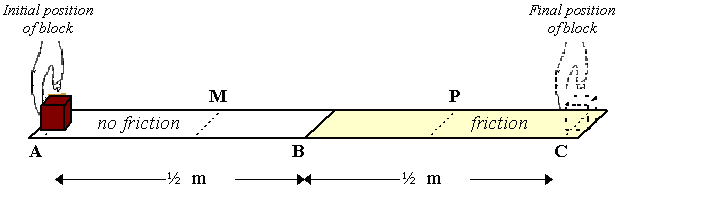Teaching Physics with the Physics Suite Edward F. Redish

Pushing a block

A hand pushes a 3 kg block along a table from point A to point C as shown in the figure below. The table has been prepared so that the left half of the table (from A to B) is frictionless. The right half (from B to C) has a non-zero coefficient of friction equal to m. The hand pushes the block from A to C using a constant force of 5 N. The block starts off at rest at point A and comes to a stop when it reaches point C. The distance from A to B is ˝ meter and the distance from B to C is also ˝ meter.1. Describe in words the motion of the block as it moves from A to C.
2. Draw a free-body diagram for the block when it is at point P.
3. What is the direction of the acceleration of the block at point P? If it is 0, state that explicitly. Explain your reasoning.
4. Does the magnitude of the acceleration increase, decrease, or remain the same as the block moves from B to C? Explain your reasoning.
5. What is the net work done on the object as it moves from A to B? From B to C?
6. Calculate the coefficient of friction m.

Note to instructor: This is a problem that attempts to help students integrate their ideas about forces with the concepts of work and energy. Many students (even physics grad students) keep these concepts separate. Giving parts (b) and (f) on an exam can produce worse results than just giving part (f). See M. Sabella, “Using the context of physics problem solving to evaluate the coherence of student knowledge”, Ph.D. thesis, University of Maryland, 1999.

Not finding what you wanted? Check the Site Map for more information.